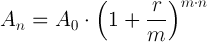# Compound interest free online calculation

Compound interest calculation formula with examples.

## Compound interest calculation formula

### Future value calculation

The future amount after n years An is equal to the initial amount A0 times one plus the annual interest rate r divided by the number of compounding periods in a year m raised to the power of m times n:An is the amount after n years (future value).

A0 is the initial amount (present value).

r is the nominal annual interest rate.

m is the number of compounding periods in one year.

n is the number of years.

#### Example #1:

Calculate the future value after 10 years present value of \$5,000 with annual interest of 4%.

Solution:

A0 = \$5,000

r = 4% = 4/100 = 0.04

m = 1

n = 10

A10 = \$5,000·(1+0.04/1)(1·10) = \$7,401.22

#### Example #2:

Calculate the future value after 8 years present value of \$35,000 with annual interest of 3% compounded monthly.

Solution:

A0 = \$35,000

r = 3% = 3/100 = 0.03

m = 12

n = 8

A8 = \$35,000·(1+0.03/12)(12·8) = \$44,480.40

Compound interest calculator »

Currently, we have around 935 calculators, conversion tables and usefull online tools and features to make your life easier or simply help you to do your work or duties faster and in more effective way. These below are the most commonly used by many users at all

And we are still developing more. Our goal is to become the one-stop, go-to site for people who need to make quick calculations or who need to find quick answer for basic conversions.

Additionally, we believe the internet should be a source of free information. Therefore, all of our tools and services are completely free, with no registration required. We coded and developed each calculator individually and put each one through strict, comprehensive testing. However, please inform us if you notice even the slightest error – your input is extremely valuable to us. While most calculators on Justfreetools.com are designed to be universally applicable for worldwide usage, some are for specific countries only.